# Using the MortalityTables Package

#### 2018-03-30

The MortalityTables package provides the mortalityTable base class and some derived classes to handle diffferent types of mortality tables (also called life tables), mainly used for life insurance. Additionally it provides a plot function to compare multiple life tables either directly using the absolute mortalities in log-linear plots or using relative mortalities as percentages of a given reference table.

## Types of Life Tables

Provided types of mortality tables are:

• Base class
Class mortalityTable
• Period life table
Class mortalityTable.period (ages, deathProbs, ..., baseYear=2000)
Death probabilities observed / predicted for one observation year; No dependency on the bith year is assumed.
• Cohort life table using age-specific trends
Class mortalityTable.trendProjection
Death probabilities of a given base year are projected into the future using age-specific trends $$\lambda_x$$. The death probability of an $$x$$-year old in year baseYear + n is calculated as: $q_x^{(baseYear+n)} = q_x^{(baseYear)} \cdot e^{-n\cdot\lambda_x}$
Consequently, the death probabilities for a person born in year YOB can be calculated as $q_x^{YOB} = q_x^{(base)} \cdot e^{-(YOB+x-baseYear)\cdot \lambda_x}$
• Cohort life table approximation using age shift
Class mortalityTable.ageShift
Death probabilities for cohort $$YOB$$ are obtained by using death probabilities for cohort $$X$$ and modifying the technical age with a birth-year dependent shift: $q_x^{YOB} = q_{x+shift(YOB)}^{(base)}$
• Mixed life table
Class mortalityTable.mixed
Arithmetic mean of two life tables with given weights. This approach is often used to generate unisex life tables by mixing male and female mortalities with given weights (e.g. 70:30 or 40:60)
• Cohort life table using age-specific improvement factors
Class mortalityTable.improvementFactors
Project base life table using age-specific improvement factors.
• Pension tables
Class pensionTable
Transition probabilities for a four-state pension model (active, invalid, retirement and death, with a possible widow in the event of death).

library("MortalityTables")

## Provided Data Sets

The package provides several real-life life tables published by census bureaus and actuarial associations around the world. You can use the function mortalityTables.list to list all available datasets (if no argument is given) or all datasets that match the given pattern (wildcard character is *). You can then use mortalityTables.load to load either one single data set or all datasets that match the pattern.

# list all available data sets
mortalityTables.list()
#>   "Austria_Annuities"
#>   "Austria_Annuities_AVOe1996R"
#>   "Austria_Annuities_AVOe2005R"
#>   "Austria_Annuities_EROMF"
#>   "Austria_Annuities_RR67"
#>   "Austria_Census"
#>   "Germany_Annuities"
#>   "Germany_Annuities_DAV1994R"
#>  "Germany_Annuities_DAV2004R"
#>  "Germany_Census"
#>  "Germany_Endowments"
#>  "Germany_Endowments_DAV1994T"
#>  "Germany_Endowments_DAV2008T"
#>  "USA_Annuities"
#>  "USA_Annuities_1971IAM"
#>  "USA_Annuities_1983a"
#>  "USA_Annuities_1994GAR"
#>  "USA_Annuities_2012IAM"
#>  "USA_Annuities_Annuity2000"

# list all datasets for Austria
mortalityTables.list("Austria_*")
#>  "Austria_Annuities"
#>  "Austria_Annuities_AVOe1996R"
#>  "Austria_Annuities_AVOe2005R"
#>  "Austria_Annuities_EROMF"
#>  "Austria_Annuities_RR67"
#>  "Austria_Census"

# Load the German annuity table DAV 2004-R

# Load all Austrian data sets
#> Loading table dataset 'Austria_Endowments_ADSt2426_2Lives'

In the next few sections we will always use some of the provided life tables for demonstration purposes.

## Working with life table objects

### Plotting life tables

The package provides several functions to plot lifetables:

• plotMortalityTables(table1, table2, ...)
A log-linear plot comparing all given life tables.
• plotMortalityTableComparisons(table1, table2, ..., reference=reftable)
Plot the given life tables as percentages relative to the reference table
• plotMortalityTrend(table1, table2, ..., YOB, Period)
Plot the yearly mortality improvement factors (for either the given observation year Period or the birth-year YOB)

These functionalities are also combined into the S3 plot function for the mortalityTable class, so you can usually just call plot on the mortality tables. If the trend = TRUE argument is given, plotMortalityTrend is used, if the reference argument is given, plotMortalityTableComparisons is used, otherwise plotMortalityTables is called.

# Log-linear plot comparing some Austrian census tables
plot(mort.AT.census.1951.male, mort.AT.census.1991.male,
mort.AT.census.2001.male, mort.AT.census.2011.male,
legend.position = c(1,0))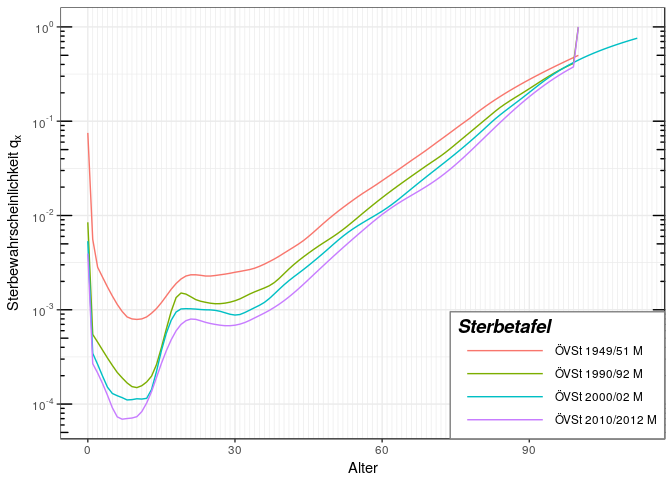# Relative death probabilities in percentage of the latest census
plot(mort.AT.census.1951.male, mort.AT.census.1991.male,
mort.AT.census.2001.male,
reference = mort.AT.census.2011.male, legend.position = c(1,0.75), ylim = c(0,4))
#> Warning in normalize_deathProbabilities(cbind(x = ages(t), y =
#> deathProbabilities(t, : Reference mortality table does not contain ages
#> 101102103104105106107108109110111112 required for normalization. These ages
#> will not be normalized!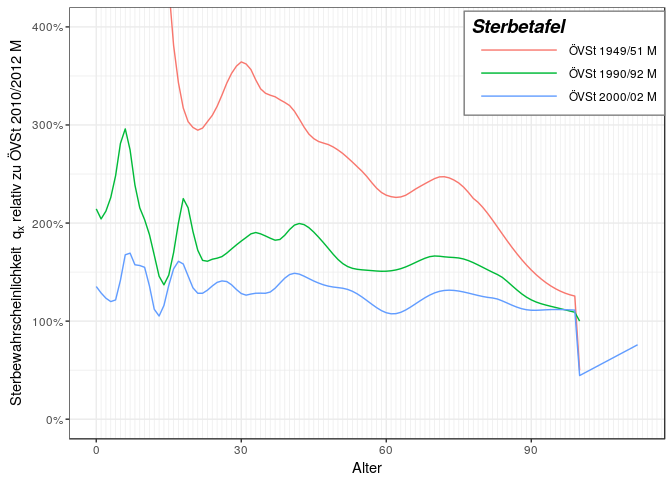For cohort life tables, the plot functions also take either the YOB or the Period parameter to plot either the cohort death probabilities for the given birth year or the period death probabilities for the given observation year.

# Comparison of two Austrian annuity tables for birth year 1977
plot(AVOe1996R.male, AVOe2005R.male, YOB = 1977, title = "Comparison for YOB=1977")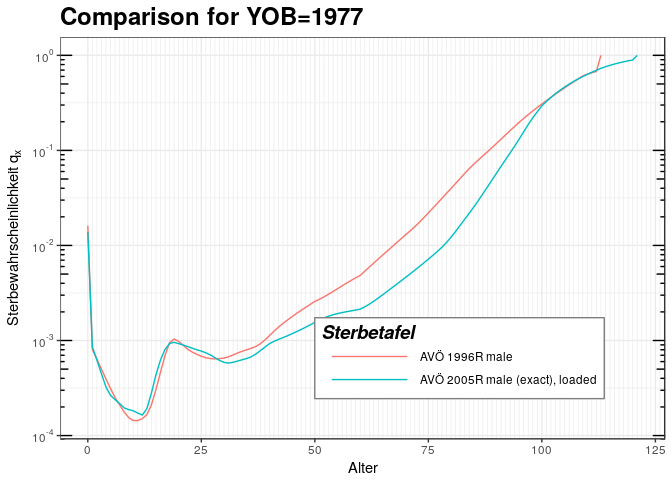# Comparison of two Austrian annuity tables for observation year 2020
plot(AVOe1996R.male, AVOe2005R.male, Period = 2020, title = "Comparison for observation year 2020")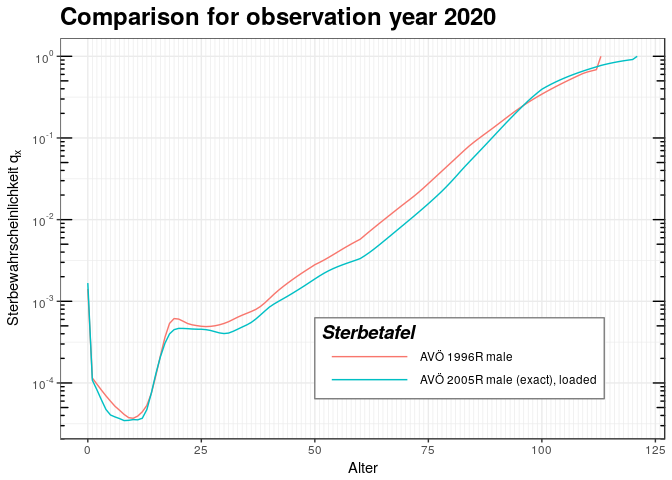### Obtaining period and cohort death probabilities

To obtain death probabilities from all the different types of tables, there are two functions:

• deathProbabilities: Returns the (cohort) death probabilities of the life table given the birth year
• periodDeathProbabilities: Returns the (period) death probabilities of the life table for a given observation year
mortalityTables.load("Austria_Annuities")
# Get the cohort death probabilities for Austrian Annuitants born in 1977:
qx.coh1977 = deathProbabilities(AVOe2005R.male, YOB = 1977)

# Get the period death probabilities for Austrian Annuitants observed in the year 2020:
qx.per2020 = periodDeathProbabilities(AVOe2005R.male, Period = 2020)

These functions return the death probabilities as a simple, numeric R vector.

There are two similar functions that return the death probabilities as a period life table object that can be used with all other functions provided by this package:

• getCohortTable: Get a mortalityTable object describing the death probabilities for people born in the given year
• getPeriodTable: Get a mortalityTable object describing the death probabilities observed in the given year
# Get the cohort death probabilities for Austrian Annuitants born in 1977 as a mortalityTable.period object:
table.coh1977 = getCohortTable(AVOe2005R.male, YOB = 1977)

# Get the period death probabilities for Austrian Annuitants observed in the year 2020:
table.per2020 = getPeriodTable(AVOe2005R.male, Period = 2020)

# Compare those two in a plot:
plot(table.coh1977, table.per2020, title = "Comparison of cohort 1977 with Period 2020", legend.position = c(1,0))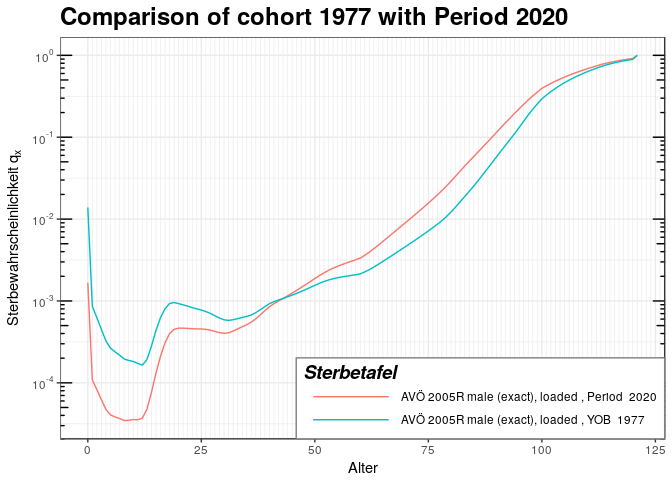Not surprisingly, at 43 years the two death probabilities cross, because in 2020 the person born 1977 is 43 years old, so the $$q_x$$ refer to the same person. However, for younger ages, the period 2020 probabilities are lower, because the mortality improvement for those younger ages has much less time in the cohort 1977 table. For ages above 43 the cohort table describes the mortality further into the future than 2020, so there is more improvement and thus lower death probabilities for the cohort life table.

### Other data extraction functions from life tables

function description
ages(table) Returns the vector of ages, for which the life table can provide death probabilities
getOmega(table) Returns the maximum age, for which the life table can provide dath probabilities
ageShift(table, YOB) Returns the age shift for the given year of birth
baseTable(table) Returns the base table, from which the table projects (for cohort tables)
baseYear(table) Returns the year of the base table
lifetable(table, YOB) Returns the cohort death probabilities as a lifetable object for use with the lifecontingencies package

## Creating a life table object

### Period life tables

Period death probabilities are the simplest type of life table, giving the probabilities of death observed during the corresponding year (the “period”). The death probabilities of different ages refer to different persons, being of the corresponding ages in the observation year. All that is needed to create a period life table are the death probabilities and the corresponding ages:

lt = mortalityTable.period(name = "Sample period lifetable", ages = 1:99, deathProbs = exp(-(99:1)/10))
plot(lt, title = "Simple log-linear period mortality table")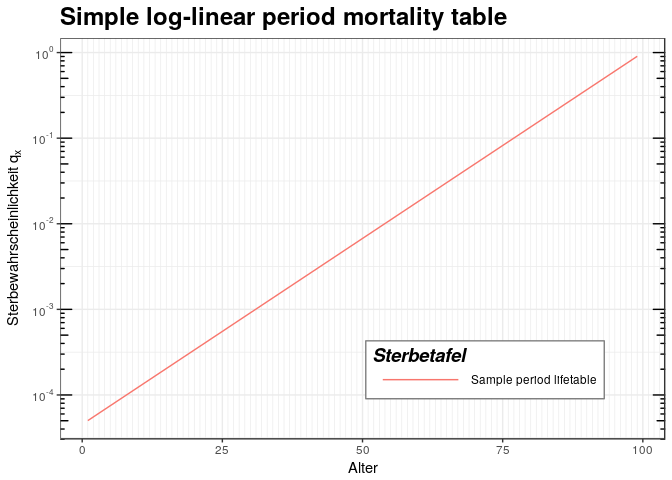deathProbabilities(lt)
#>   5.017468e-05 5.545160e-05 6.128350e-05 6.772874e-05 7.485183e-05
#>   8.272407e-05 9.142423e-05 1.010394e-04 1.116658e-04 1.234098e-04
#>  1.363889e-04 1.507331e-04 1.665858e-04 1.841058e-04 2.034684e-04
#>  2.248673e-04 2.485168e-04 2.746536e-04 3.035391e-04 3.354626e-04
#>  3.707435e-04 4.097350e-04 4.528272e-04 5.004514e-04 5.530844e-04
#>  6.112528e-04 6.755388e-04 7.465858e-04 8.251049e-04 9.118820e-04
#>  1.007785e-03 1.113775e-03 1.230912e-03 1.360368e-03 1.503439e-03
#>  1.661557e-03 1.836305e-03 2.029431e-03 2.242868e-03 2.478752e-03
#>  2.739445e-03 3.027555e-03 3.345965e-03 3.697864e-03 4.086771e-03
#>  4.516581e-03 4.991594e-03 5.516564e-03 6.096747e-03 6.737947e-03
#>  7.446583e-03 8.229747e-03 9.095277e-03 1.005184e-02 1.110900e-02
#>  1.227734e-02 1.356856e-02 1.499558e-02 1.657268e-02 1.831564e-02
#>  2.024191e-02 2.237077e-02 2.472353e-02 2.732372e-02 3.019738e-02
#>  3.337327e-02 3.688317e-02 4.076220e-02 4.504920e-02 4.978707e-02
#>  5.502322e-02 6.081006e-02 6.720551e-02 7.427358e-02 8.208500e-02
#>  9.071795e-02 1.002588e-01 1.108032e-01 1.224564e-01 1.353353e-01
#>  1.495686e-01 1.652989e-01 1.826835e-01 2.018965e-01 2.231302e-01
#>  2.465970e-01 2.725318e-01 3.011942e-01 3.328711e-01 3.678794e-01
#>  4.065697e-01 4.493290e-01 4.965853e-01 5.488116e-01 6.065307e-01
#>  6.703200e-01 7.408182e-01 8.187308e-01 9.048374e-01

### Cohort life tables with trend projection

A cohort life table with trend projection needs the following parameters:

• The base table $$q_x^{(base)}$$ (death probabilities) for the given base period as a vector
• Age-specific trend factors $$\lambda_x$$ as a vector
• The base year (numeric)
atPlus2 = mortalityTable.trendProjection(
name = "Austrian Census Males 2011, 2% yearly trend",
baseYear = 2011,
deathProbs = deathProbabilities(mort.AT.census.2011.male),
ages = ages(mort.AT.census.2011.male),
trend = rep(0.02, length(ages(mort.AT.census.2011.male)))
)

Some life tables do not assume a constant age-specific trend over time, but rather assume that the currently observed high mortality improvements are just a temporary effect, so the current trend is in effect only for some time and then reduces to some kind of long-term trend.

There are two conceptual approaches: One is to use a trend dampening function that is simply applied to the starting trend. So, while the initial trend might be 3%, i.e. the projection will use (ObservationYear-BaseYear) * OriginalYear, over time it will assume the value dampeningFunction(ObservationYear-BaseYear) * OriginalTrend. The dampening function in this case gives the cumulated trend effect from the base year until the observation year. To implement this trend reduction with the MortalityTables package, simply pass a one-argument function as the dampingFunction slot to the class, the argument will be the number of years from the base year (NOT the calendar year!):

atPlus2.damp = mortalityTable.trendProjection(
name = "Austrian M '11, 2% yearly, damping until 2111",
baseYear = 2011,
deathProbs = deathProbabilities(mort.AT.census.2011.male),
ages = ages(mort.AT.census.2011.male),
trend = rep(0.02, length(ages(mort.AT.census.2011.male))),
# damping function: 2011: full effect, linear reduction until yearly trend=0 in 2111:
# 2011: 100%, 2012: 99%, 2013: 98% => For 2013 we have a cumulative trend
# of 297% instead of 300% for three full yearly trends!
dampingFunction = function(n) { n - n * (n + 1) / 2 / 100 }
)

plot(mort.AT.census.2011.male, atPlus2, atPlus2.damp, YOB = 2011, legend.position = c(0.8,0.75))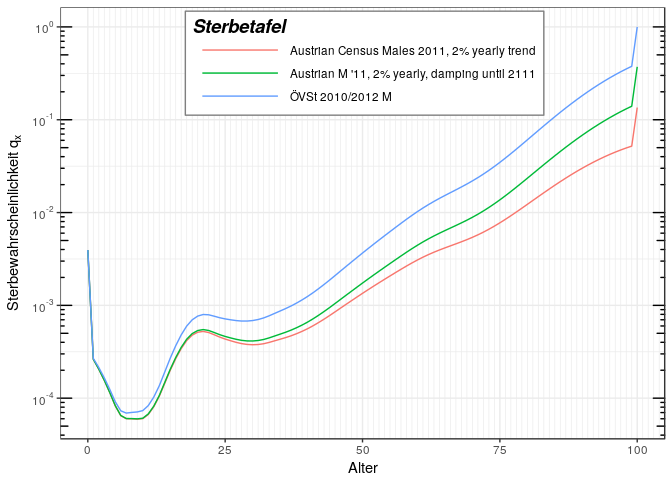The other approach is to assume that instead of the initial trend, after some time a second trend (slot trend2) takes over. In this case, the dampingFunction slot is again a one-argument function that now gives the weight of the first trend, while 1-dampingFunction(year) will give the weight of the second trend. As the weights will be applied for the whole period from the base- to the observation year, the weights need to be cumulated and normalized.

The argument in this case is the actual calendar year (not the year since the base year like it was in the one-trend case above!)

atPlus2.damp2 = mortalityTable.trendProjection(
name = "Austrian M '11, 2% yearly, 1% long-term",
baseYear = 2011,
deathProbs = deathProbabilities(mort.AT.census.2011.male),
ages = ages(mort.AT.census.2011.male),
trend = rep(0.02, length(ages(mort.AT.census.2011.male))),
trend2 = rep(0.01, length(ages(mort.AT.census.2011.male))),
# damping function interpolates between the two trends:
# until 2021 trend 1, from 2031 trend 2, linearly beteen
dampingFunction = function(year) {
if (year <= 2021) 1
else if (year > 2031) 14.5/(year - 2011)
else 1 - (year - 2021)*(year - 2021 + 1) / 20 / (year - 2011)
}
)

plot(mort.AT.census.2011.male, atPlus2, atPlus2.damp, atPlus2.damp2, YOB = 2011, legend.position = c(0.8,0.75))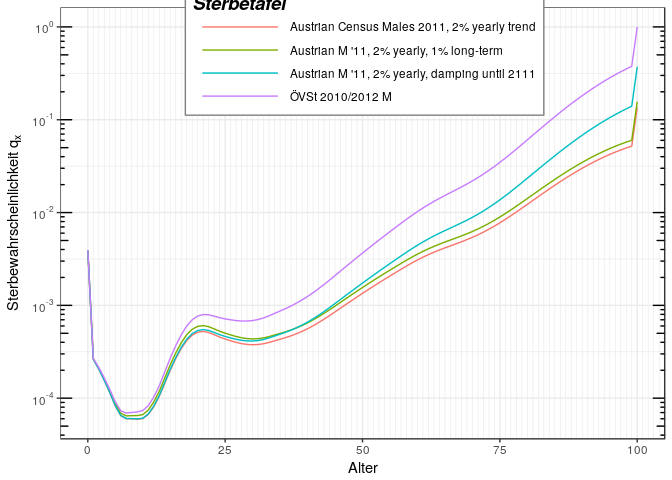### Cohort life tables with age-shift

Age-shifted cohort life tables are an approximation to full cohort life tables. Full cohort life tables apply a trend or improvment factors to the death probabilities of a base year to obtail death probabilities for a given birth year. Age-shifting rather modifies the age of the corresponding person and uses the same, unmodified base table for all cohorts. Basically, it works like this:

A 60-year old born in 1950 has the same death probability as a 50-year old born in 1900, so instead of looking at the cohort 1950, we can look at the cohort 1900 and for a person born 1950 we treat him as if he were 10 years younger.

So, an age-shifted cohort life table just needs the base table and for each birth year the amount the age is modified.

For those people, who think visually, age shifting works on the death probabilities as following: A normal trend moves the $$q_x$$ curve downwards. Age-shifting approximates this by shifting the $$q_x$$ curve to the right without modifying its values.

The following example clearly shows this, with the blue curve being the base table for YOB 2011. A full trend projection moves the curve down to the green line, while age-shifting moves the base curve to the right so that it coincides as much as possible with the exact (green) line.

baseTableShift = getCohortTable(atPlus2, YOB = 2011);
baseTableShift@name = "Base table of the shift (YOB 2011)"

atShifted = mortalityTable.ageShift(
name = "Approximation with age shift",
baseYear = 2011,
deathProbs = deathProbabilities(baseTableShift),
ages = ages(baseTableShift),
ageShifts = data.frame(
shifts = c(
rep( 0, 3),
rep(-1, 3),
rep(-2, 3),
rep(-3, 3),
rep(-4, 3),
rep(-5, 3),
rep(-6, 3)
),
row.names = 2011:2031
)
)

ageShift(atShifted, YOB = 2021)
#>  -3

plot(baseTableShift, atPlus2, atShifted, YOB = 2021, legend.position = c(0.8,0.75))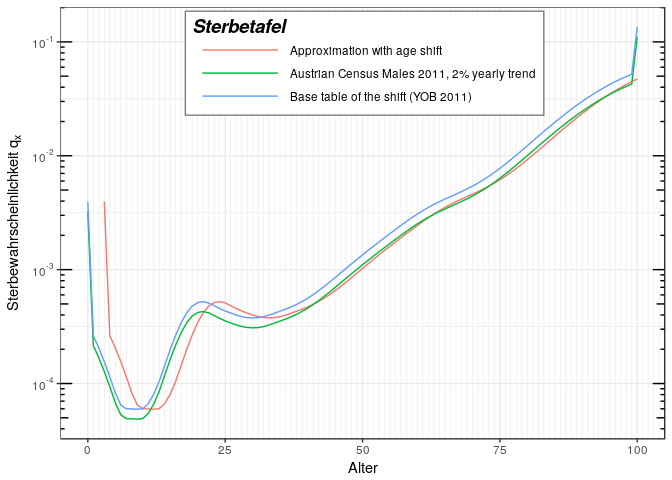As one can see, for ages above 40 years, the table with 2% yearly trend and the corresponding age-shifted table have roughly the same mortalities. Below 40 years, the two are very different, so this approximation through age-shifting should really be used with extreme care!

## Modifying life table objects

### Copying life tables

Life tables are simple pass-by-value S4 objects, so copying works by simple assignment.

b = AVOe2005R.female
b@name = "Modified Copy"
# only b is modified, not the original table
b@modification = function(qx) pmax(qx, 0.01)
plot(AVOe2005R.female, b, YOB = 2000)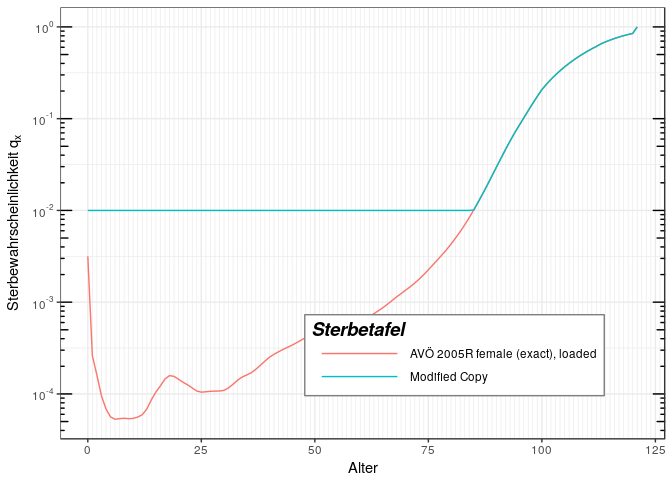When calculating premiums for life insurance contracts, one often needs to add a certain security loading on the raw death probabilities (e.g. 10% increased death probabilities) to account for statistical fluctuations. This can be easily done with the setLoading function that returns a copy of the given table and adds the given security loading.

AVOe2005R.female.sec = setLoading(AVOe2005R.female, loading = 0.1);
# Make sure the modified table has a new name, otherwise plots might break
plot(AVOe2005R.female, AVOe2005R.female.sec, title = "Original and modified table")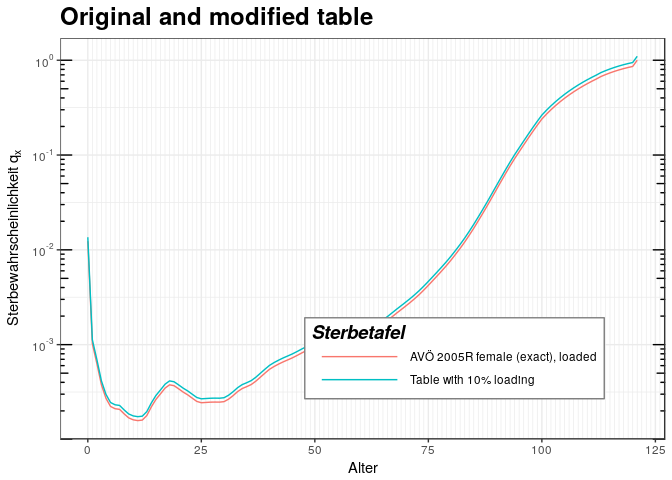### Adding a modification to the raw probabilities

Some uses require post-processing of the death probabilities, like adding a lower bound for the death probabilities. To achive this, all mortalityTable-derived classes have a slot modification that takes a function that is passed the vector of death probabilities.

AVOe2005R.female.mod = setModification(AVOe2005R.female, modification = function(qx) pmax(0.03, qx));
# Make sure the modified table has a new name, otherwise plots might break
AVOe2005R.female.mod@name = "Modified table (lower bound of 3%)"
plot(AVOe2005R.female, AVOe2005R.female.mod, title = "Original and modified table")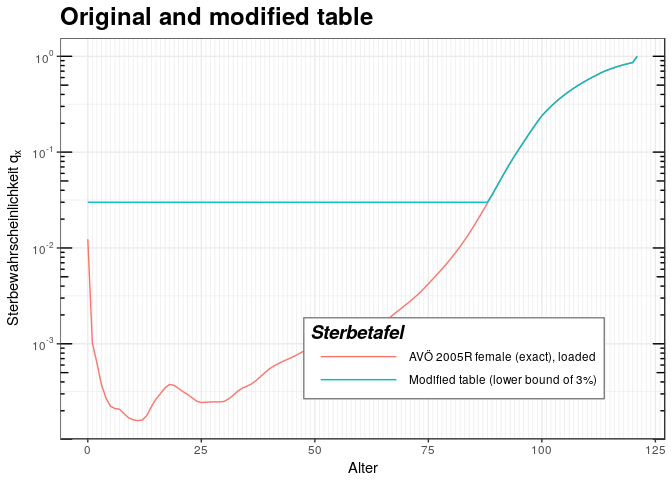## Pension Tables

Pension tables generalize mortality tables in that the state space is increased from two states (alive / dead) to four states (active / invalidity or realy retirement / old age retirement / dead). As a consequence, there is no longer just one transition probability, but multiple.

Possible states are: * active: healty, no pension, typically paying some kin of premium * incapacity: disablity pension (in most cases permanent), not working, early pension * retirement: old age pension, usually starting with a fixed age * dead * Widow/widower pension (if a widow exists at the time of death)

Correspondingly, the pensionTable class offers the following slots describing transition probabilities for the corresponding state transitions (by a mortalityTable-derived object): * qxaa: death probability of actives (active -> dead) * ix: invalidity probability (active -> incapacity) * qix: death probability of invalid (invalid -> dead) * rx: reactivation probability (incapacity -> active) * apx: retirement probability (active -> retirement), typically 1 for a fixed age * apx: retirement probability of invalids (invalid -> retirement), typically 0 or 1 for a fixed age * qpx: death probability of retired (retired -> dead) * hx: probability of a widow at moment of death (dead -> widow), y(x) age differene * qxw: death probability of widows/widowers * qgx: death probability of total group (irrespective of state)

All functions that handle mortalityTable object can be used with these slots.

Additionally, the following functions are provided to obtain the set of all transition probabilities in one data frame: * transitionProbabilities(pension_table, YOB) * periodTransitionProbabilities(pension_table, Period)